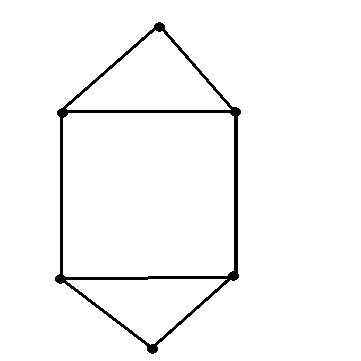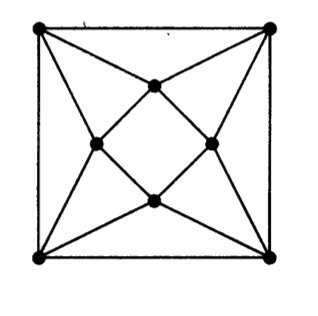MTH4140 Home

# MTH4140: Midterm 2 Practice 1

Problem 1

Prove that for every positive integer $$k$$ there exists a graph $$G$$ such that $$\chi(G)=\Delta(G)-k$$.

Problem 2

Determine the chromatic polynomial of the graph below.Problem 3

Determine the $$\chi(\mathcal G)$$ for the graph $$\mathcal G$$ below and a $$\chi(\mathcal G)$$-critical subgraph.Problem 4

Let $$G_n=(V_n,E_n)$$ be a graph where $$V_n=\{1,2,\dots, n\}$$ and $$E_n$$ is defined in the following way: $$(i,j)\in E_n$$ if and only if $$i+j$$ is not divisible by $$3$$. Find the chromatic number of $$G_n$$.

Problem 5

Find all integers $$n$$ for which there is a graph $$G$$ such that $$\omega(G)=2$$ and $$\chi(G)=n$$.